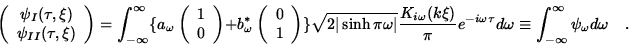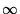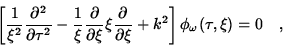Next: Geometry of the Space Up: ACCELERATION-INDUCED CARRIER OF THE Previous: ACCELERATION-INDUCED CARRIER OF THE

## Relativistic Quantum Mechanics

Our starting point is non-trivial relativistic quantum mechanics as expressed by the Klein-Gordon equation1

where k2=k2x +k2y +m2 .

The objective is to deduce from this equation a carrier of the imprints of gravitation with the following three fundamental requirements:

1. The imprints must be carried by the evolving dynamics of a quantum mechanical wavefunction.

2. Even though the dynamical system is characterized by its mass m , the carrier and the imprints must not depend on this mass, i.e. the carrier must be independent of k2 . This requirement is analogous to the classical one in which the world line of a particle is independent of its mass.

3. In the absence of gravitation the carrier should yield measurable results (expectation values) which are invariant under Lorentz boosts and spacetime translations.

We shall now expand on these three requirements. In quantum mechanics the wave function plays the role which in Newtonian mechanics is played by a particle trajectory or in relativistic mechanics by a particle world line. That the wave function should also assume the task of carrying the imprints of gravitation is, therefore, a reasonable requirement.

Because of the Braginski-Dicke-Eotvos experiment, the motion of bodies in a gravitational field is independent of the composition of these bodies, in particular their mass. Consequently, the motion of free particles in spacetime traces out particle histories whose details depend only on the gravitational environment of these particles, not on their internal constitution (uniqueness of free fall, weak equivalence principle''). Recall the superposition of different wave functions (states) of a relativistic particle yields interference fringes which do depend on the mass of a particle (incompatibility between quantum and equivalence principle'' ). If the task of these wave functions is to serve as carriers of the imprints of gravitation, then, unlike in classical mechanics, these interfering wave functions would do a poor job at their task: They would respond to the presence (or absence) of gravitation in a way which depends on the details of the internal composition (mass) of a particle. This would violate the simplicity implied by the Braginski-Dicke-Eotvos experiment. Thus we shall not consider such carriers. This eliminates any quantum mechanical framework based on energy and momentum eigenfunctions because the dispersion relation, E2=m2 +p2z +p2y +p2x , of these waves depends on the internal mass m .

Recall that momentum and energy are constants of motion which imply the existence of a locally inertial reference frame. Consequently, requirement 2. rules out inertial frames as a viable spacetime framework to accomodate any quantum mechanical carrier of the imprints of gravitation. Requirement 2. also rules out a proposal to use the interference fringes of the gravitational Bohm-Aharanov effect to carry the imprints of gravitation . This is because the fringe spacing depends on the rest mass of the quantum mechanical particle.

Requirement 3. expresses the fact that the quantum mechanical carrier must remain unchanged under the symmetry transformations which characterize a two-dimensinal spacetime. By overtly suppressing the remaining two spatial dimensions we are ignoring the requisite rotational symmetry. Steps towards remedying this neglect have been taken elsewhere .

We shall now exhibit a carrier which fulfills the three fundamental requirements. It resides in the space of Klein-Gordon solutions whose spacetime domain is that of a pair of frames accelerating into opposite directions (Rindler frames''). These frames partition spacetime into a pair of isometric and achronally related Rindler Sectors I and II ,2

Suppose we represent an arbitrary solution to the K-G equation in the form of a complex two-component vector normal mode expansion3

This is a correlated (entangled'') state with two independent degrees of freedom. There is the polarization degree of freedom in addition to the spatial degree of freedom. The polarization degree of freedom has a two-dimensional space of states (C2 ) spanned by two-spinors. The two components of a spinor refer to the wave amplitude at diametrically opposite events on a Cauchy hypersurfacein Rindler I and II respectively. The spatial () degree of freedom has a state space () which is-dimensional and which is spanned by the scalar boost eigenfunctions4

solutions to the Rindler wave equation5

which is the equation obtained by applying the coordinate transformation Eq.(2) to the Klein-Gordon Eq.(1).Next: Geometry of the Space Up: ACCELERATION-INDUCED CARRIER OF THE Previous: ACCELERATION-INDUCED CARRIER OF THE
Ulrich Gerlach
1998-02-20